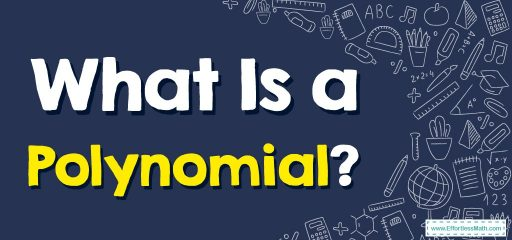# What Is a Polynomial?

A polynomial is an algebraic expression in which terms are separated using the "+" and "-" operators. In this guide, you will learn more about the definition of a polynomial and its properties.A polynomial is a kind of expression. An expression is a mathematical statement without an equal-to sign $$(=)$$.

## A step-by-step guide topolynomial

A polynomial is a type of algebraic expression in which the exponents of all variables must be a whole number. The power of variables in any polynomial must be a non-negative integer. A polynomial contains constants and variables, but we can not perform division operations by a variable in polynomials.

The following image shows all the terms in a polynomial:

### Terms of a polynomial

Polynomial terms are defined as parts of an expression that are separated by the operators $$+$$ or $$-$$. For example, the polynomial expression $$5x^3-\:4x^2+\:8x\:-12$$ consists of four terms.

### Degree of a polynomial

The highest or greatest power of a variable in a polynomial is known as the degree of the polynomial. The degree is used to determine the maximum number of solutions of a polynomial equation.

Note: The degree of a polynomial with more than one variable is equal to the sum of the power of the variables in it.

### Types of polynomials

Polynomials can be categorized based on their degree and power. Based on the number of terms, there are mainly three types of polynomials listed below:

• Monomials
• Binomials
• Trinomials

A monomial is a type of polynomial with a single term. For example, $$x$$, $$-4xy$$, and $$7z^2$$. A binomial is a type of polynomial that has two terms. For example, $$x + 3$$, $$y^2+5$$, and $$2x^3- 6$$. While a trinomial is a type of polynomial that has three terms. For example $$3x^2+ 7x -5$$, $$x + y + z$$, and $$4x + y- 8$$.

### What people say about "What Is a Polynomial? - Effortless Math: We Help Students Learn to LOVE Mathematics"?

No one replied yet.

X
30% OFF

Limited time only!

Save Over 30%

SAVE $5 It was$16.99 now it is \$11.99